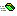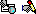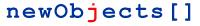HashCryptStreams Symmetric object (symmetric algorithms)

### Creation and ID-s

Program ID: newObjects.crypt.Symmetric
ClassID: {10C0E590-78A0-426A-9C78-64DEA486B982}
ProgramID: newObjects.crypt.Symmetric.free
ClassID: {00F7D232-E452-406a-A8EF-5B4116E02C4E}

Object rference

### Overview

This object provides access to symmetric encryption and encoding algorithms. The symmetric algorithms work with blocks with different sizes. For example DES uses 8 byte blocks. This is so because many of the symmetric algorithms are actually complex permutations of the data in the block. When the algorithm does not use blocks the block size is assumed 1 byte which enables us to consider these non-block based algorithms as block algorithms.

Because of this nature of the symmetric algorithms you cannot always just pass the data and get the result. The data must fit complete block in order to be processed. Thus when it does not it must be padded with several bytes. To cope with this requirement the object supports internal buffers in which the remaining data that is kept until more data is supplied or the operation is finalized. When the operation is finalized the object automatically pads the remaining data and completes the encryption returning the last chunk(s) of encrypted data. The automatic padding is controlled by the PadType property (see the notes about it in the members reference.).

Notice the Encrypt and Decrypt methods below. They have second optional parameter bFinalize. By default it is True which means that by default if you pass only the data parameter all the data is encrypted at once. If needed it is padded and the result is returned. Thus each call to Encrypt/Decrypt is completely independent this way which makes this the simplest way to use the object.

An example:

```Set crypt = Server.CreateObject("newObjects.crypt.Symmetric")
Set sf = Server.CreateObject("newObjects.utilctls.SFMain")
Set file = sf.OpenFile("somefiletoencrypt",&H40) ' Open some file
' Init the crypt
crypt.Init "AES"
crypt.Key = "0123456789ABCDEF0123456789ABCDEF"
' Now read the whole file in single step and encrypt it.
' continue by doing something with the encrypted data.```

If you want to encrypt/decrypt huge amounts of data you would want to perform this in chunks. To do so you need to call Encrypt/Decrypt with bFinalize set to False many times and finally when the last chunk is supplied with bFinalize set to True to ensure any remaining data in the internal buffer is processed.

Lets do the same as in the above example but in chunks:

```Set crypt = Server.CreateObject("newObjects.crypt.Symmetric")
Set sf = Server.CreateObject("newObjects.utilctls.SFMain")
Set file = sf.OpenFile("somefiletoencrypt",&H40) ' Open some file
' Init the crypt
crypt.Init "AES"
crypt.Key = "0123456789ABCDEF0123456789ABCDEF"
' It will make no sense to encrypt data in chunks and keep
' it in memory. So, lets open an output file
Set outfile = sf.CreateFile("encryptedfile")
' Now read the file in cycle and write the encrypted data in the outfile.
While Not file.EOS
If Not IsEmpty(cipher) Then outfile.WriteBin cipher
Wend
cipher = crypt.Encrypt(Empty, True)
If Not IsEmpty(cipher) Then outfile.WriteBin cipher```

As you can see from the above code we can expect that Encrypt will return Empty instead of encrypted data at any time. This would happen if the data we pass is less then the block size of the specified algorithm. This may look ridiculous sometimes, especially when you know the block size and how much data is passed each call. Still, omitting the finalization or/and assuming that the data is always block size aligned can easily mislead you when some changes are made to the code. Better be punctual and guarantee that the piece of code performing the encryption/decryption will continue to work correctly even if some parameters are drastically changed.

The encrypted data is always N block sizes (N - positive integer). So, why finalize it at all? Because of the buffers and the size of the chunks you decrypt each time. Yes, you can design the code to read from the file chunks multiple of the block size, but this is often inconvenient - especially if the code is supposed to work with many different algorithms. So, the best way is to perform the decryption the same way as the encryption (just calling the Decrypt method instead of Encrypt).

### ReferenceEncrypt Syntax: v = object.Encrypt(data [,bFinalize]) Encrypts the data. The data can be binary (byte array - VT_UI1|VT_ARRAY) or a string. If it is a string the specified code page is used for conversion. bFinalize if omitted is assumed True. If set to False the data that does not fit the block size is buffered and processed later when you call this method again to pass more data and the the accumulated data size is equal or greater than the block size.  The object must be initialized (Init) and a key (Key) must be set if required by the algorithm specified.Decrypt Syntax: v = object.Decyrpt(data [,bFinalize]) Decrypts the data. The data can be binary (byte array - VT_UI1|VT_ARRAY) or a string. If it is a string the specified code page is used for conversion. bFinalize if omitted is assumed True. If set to False the data that does not fit the block size is buffered and processed later when you call this method again to pass more data and the the accumulated data size is equal or greater than the block size. The object must be initialized (Init) and a key (Key) must be set if required by the algorithm specified.Reset Syntax: object.Reset Resets the internal buffers and states if you want to cancel Encrypt/Decrypt process performed in chunks.ResetKey Syntax: object.ResetKey Resets the internal buffers, the key and states if you want to cancel Encrypt/Decrypt process performed in chunks and also change the key.Init Syntax: object.Init(alg) Initializes/Resets the object with the specified algorithm (alg). The alg parameter is a string - the name of the desired symmetric encryption algorithm. Currently supported are: AES - Advanced Encryption Standard. DES - DES or 3DES depending on the length of the key specified.BlockSize Syntax: bs = object.BlockSize Returns the block size for the specified algorithm in bytes. When you call Encrypt/Decrypt with bFinalize set to False the data that does not fit the block size is buffered and the objects does not process it until further calls are made with more data. When finalizing the application can check this property and pad the data in some application specific manner (if desired). Otherwise the data is padded automatically according to the PadType property.PadType Syntax: object.PadType = n n = object.Padtype Specifies how to pad the data if it does not fit a complete buffer size when the encryption is finalized. If set to value between 0 and 255 bytes with that value are repeated to pad the data. If -1 is specified random bytes are generated.codePage Syntax: object.CodePage = cp cp = object.CodePage Specifies the code page which will be used when textual data is encrypted. If set to -1 the data is encrypted as UNICODE text.Key Syntax: object.Key = k k = object.Key Specifies the key for the selected (with Init method) encryption algorithm. Ignored if the algorithm does not use a key (for example for an encoding algorithm). The different algorithms require specific key lengths. Here is the list: AES - The key can be 16, 24 or 32 bytes. DES - The key can be 8, 16 or 24 bytes. Note that 16 and 24 byte keys cause 3DES to be performed.

...Copyright 2001-2006 newObjects [ ]